# 9大维度全面PK！袍江四大刚需红盘，哪个是你心中C位？Round One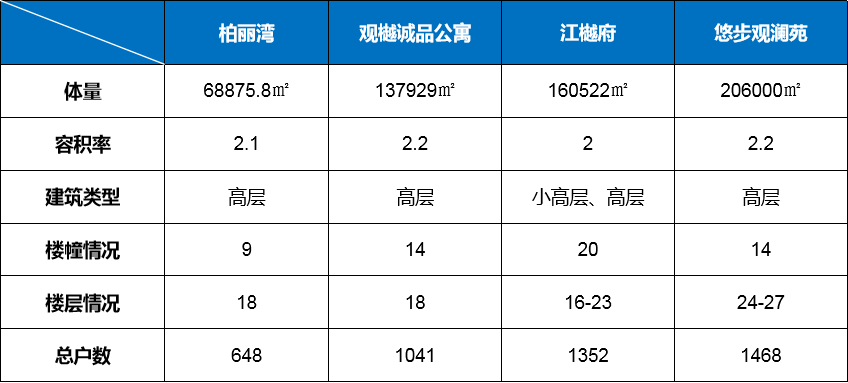Round Two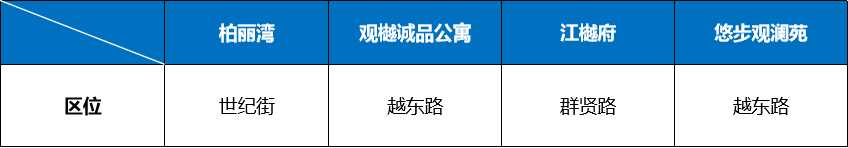Round Three

23载金辉是中国房地产开发商36强，今年上半年首入绍兴，本月又竞得镜湖凤林西路宅地，有持续深耕之意；中天美好是中国企业500强中天集团旗下的地产开发商，曾参与建设过杭州阿里中心和奥体中心等知名地标。

Round Four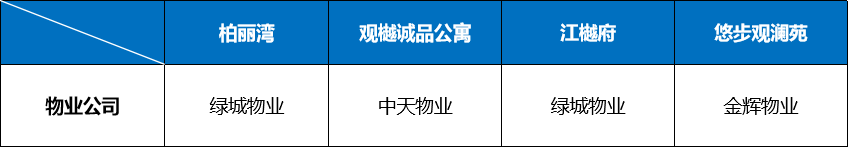Round Five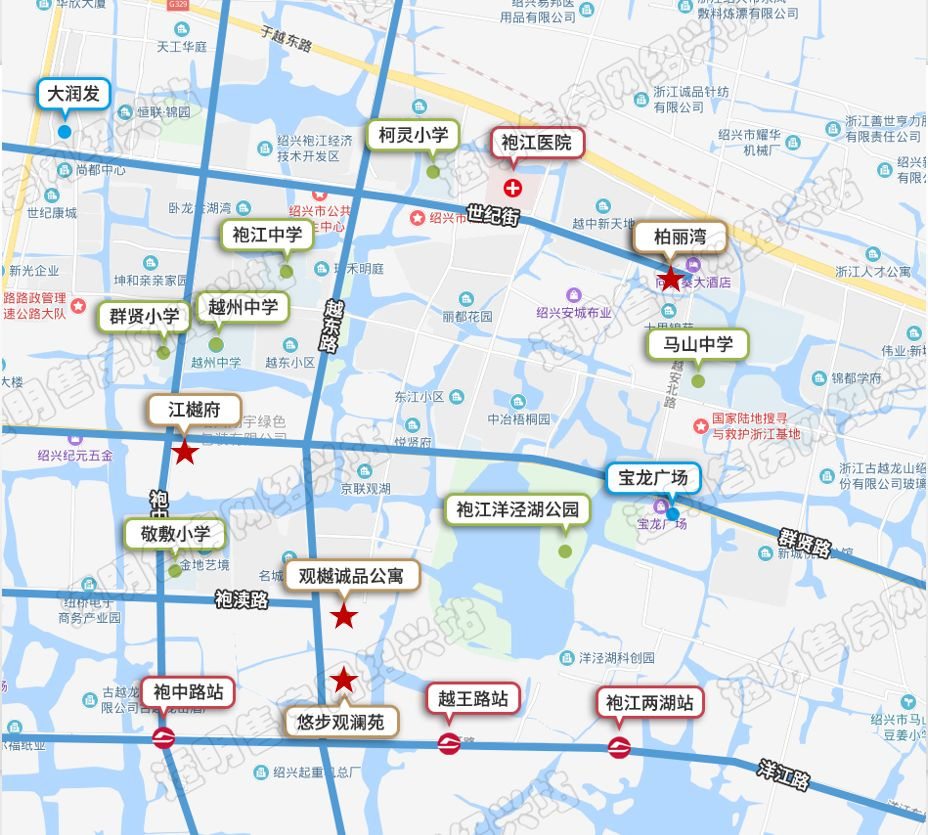Round SixRound SevenRound Eight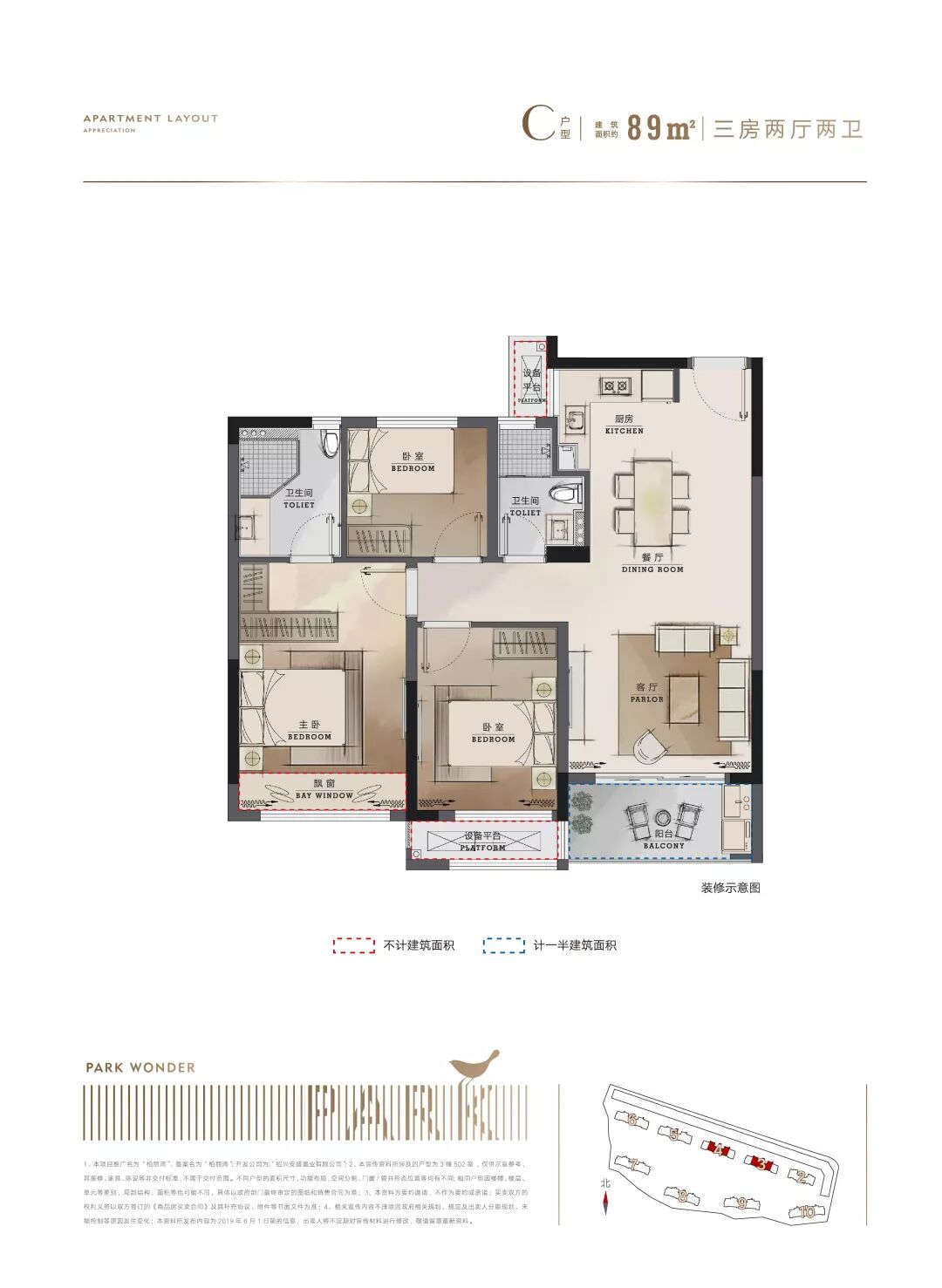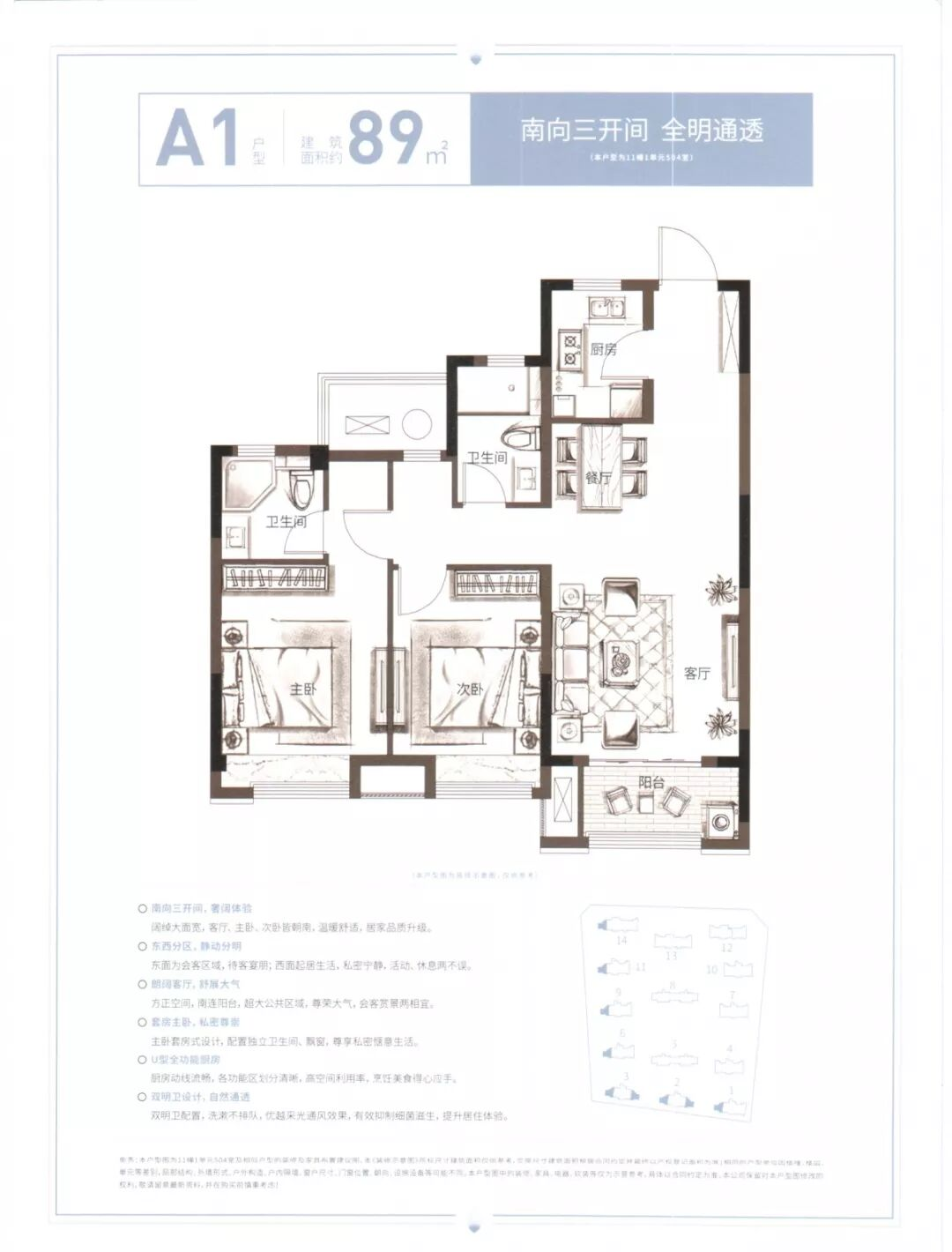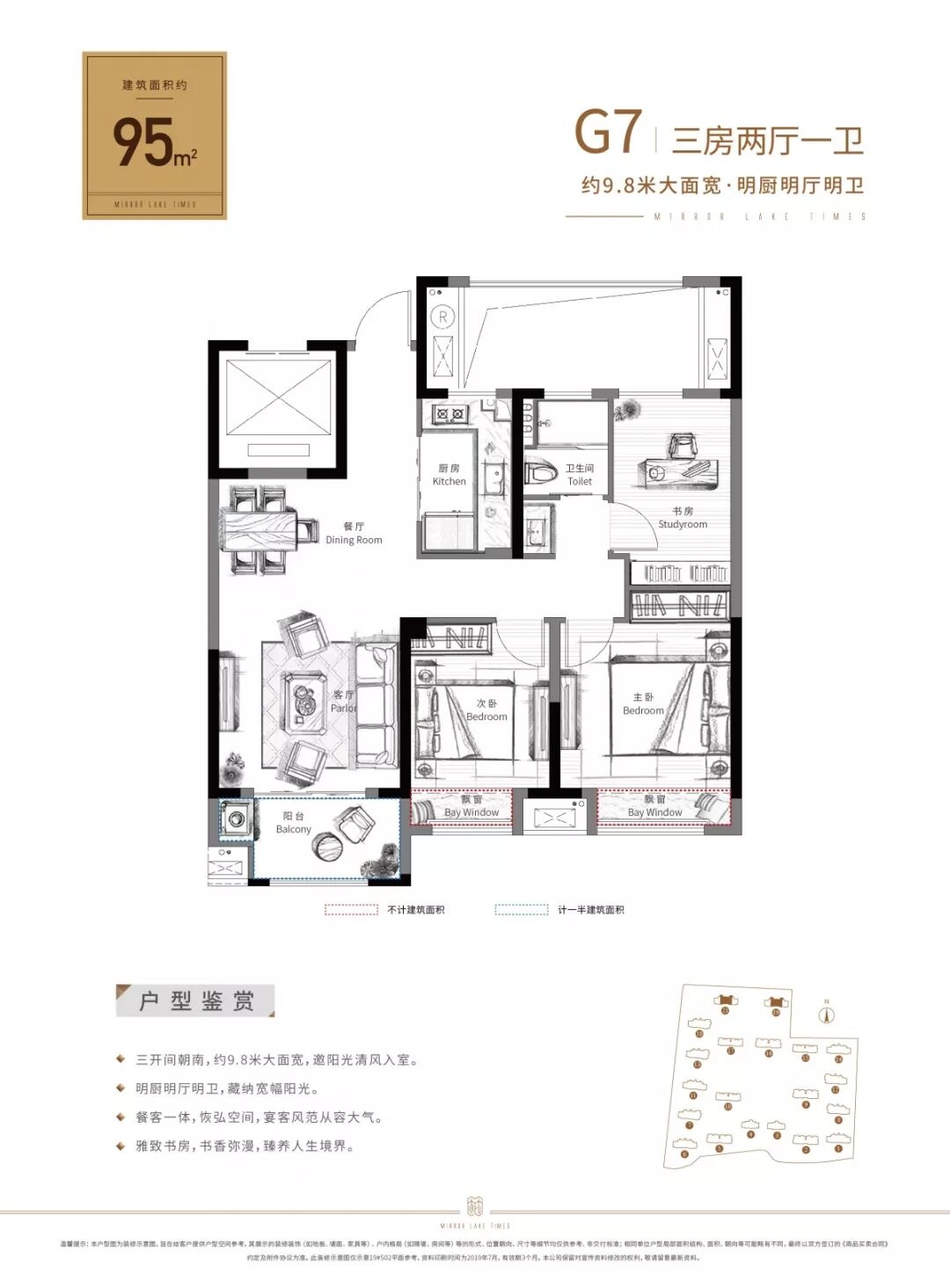Round Nine• 周边楼盘

• A
• B
• C
• D
• E
• F
• G
• H
• I
• J
• K
• L
• M
• N
• O
• P
• Q
• R
• S
• T
• U
• V
• W
• X
• Y
• Z